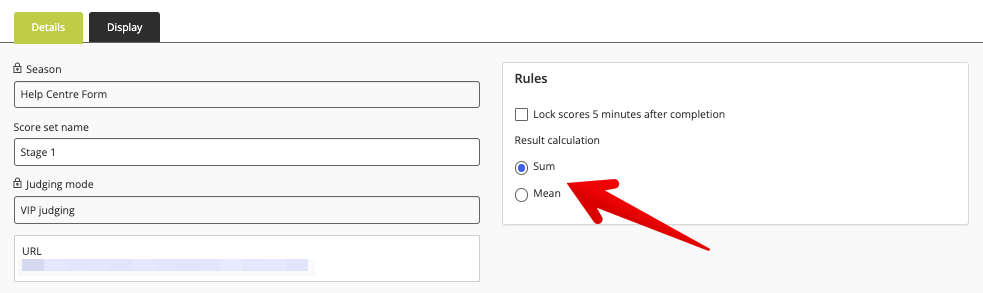# What is the difference between 'Sum' and 'Mean' result calculation?

When configuring a score set for VIP judging configuration, you will be prompted to select whether the final score will be calculated by 'Sum' or 'Mean'.Put simply, 'Sum' calculation is the total of all criteria together. 'Mean' calculation takes things one step further by dividing the total by the number of criteria. To better illustrate this, check out the example below.

Note: 'Mean' calculation is affected by the weights added to your individual scoring criteria. For a breakdown of why this is the case, see this helpful resource. If desired, you can apply a weight of 0 to your scoring criteria only if the score set calculation is set to 'Mean'.

## Example

 Sum Mean Criteria 1: 5/10 points Criteria 1: 5/10 points Criteria 2: 7/10 points Criteria 2: 7/10 points Criteria 3: 9/10 points Criteria 3: 9/10 points Total score = 21/30 points Total score = 7/10 points Formula: (5 + 7 + 9 = 21) Formula: (5 + 7 + 9 = 21 / 3 criteria = 7)

The same calculations can also be applied to criteria with differing weights.

'Sum' and 'Mean' calculations do not affect the leaderboard.

The leaderboard will always show the average score of each entry — it's the 'score out of' part which changes.

With 'Sum', it will be out of the total of all criteria.
With 'Mean', it will be out of the average of all criteria.